#### You may also like### Always Two

Find all the triples of numbers a, b, c such that each one of them plus the product of the other two is always 2.### Not Continued Fractions

Which rational numbers cannot be written in the form x + 1/(y + 1/z) where x, y and z are integers?### Surds

Find the exact values of x, y and a satisfying the following system of equations: 1/(a+1) = a - 1 x + y = 2a x = ay

# Traffic Tunnel

##### Age 14 to 16 Short Challenge Level:

Answer: None of the vehicles will fit through the tunnel.

a) truck                                        b) coach                                      c) lorry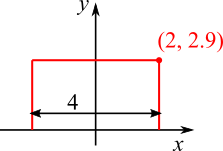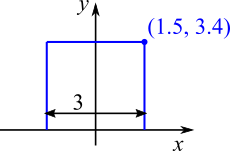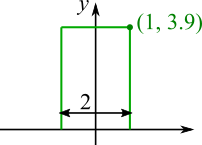How high is the tunnel at $x=1, x=1.5, x=2$?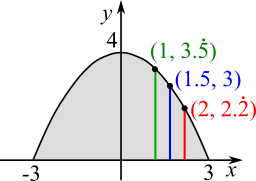$x=1\Rightarrow y=\frac49(9-1)=\frac{32}9=3\frac59=3.\dot5$

$x=1.5\Rightarrow y=\frac49(9-1.5^2)= \frac49\left(\frac{36}4-\frac94\right) = 4-1=3$

$x=2\Rightarrow y=\frac49(9-2^2)=\frac49\times5=2\frac29=2.\dot2$

All of the vehicles are too tall (or too wide) for the tunnel: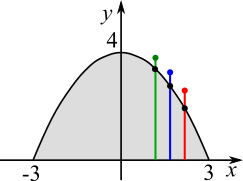$3.9\gt3.\dot5$

$3.4\gt3$

$2.7\gt2.\dot2$

You can find more short problems, arranged by curriculum topic, in our short problems collection.